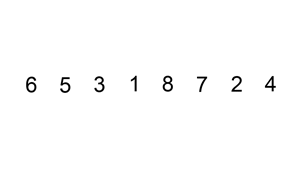Insert sort is a fast algorithm for small lists. On some languages, insert sort is used to sort small arrays (small sometimes means less than 10) instead of more complex algorithms because it’s fast enough and doesn’t need much memory.To perform Insert-sort: take one element from the list on each iteration, starting from the first, and compare with the next element. If the previous one is greater, move it one position ahead. Continue comparing the rest of the elements pushing them forward until you find a the place of the current value and no elements are left. Repeat with next element in list.

O(n2)

### Pseudo code

``````
function insert_sort(list):
(evaluate the list forward)
for i from 1 to length_of_list - 1:
value = list[i]
(evaluate the list backward from i):
while prev_position >= 0 and list[prev_position] > value:
(swap values from current position to the next)
list[prev_position+1] = list[prev_position]
go on position back in the list
if there was no swap, set value back in current position
``````

### PHP code example

``````
function insert_sort(\$list) {
for(\$i = 0; \$i < count(\$list); \$i++) {
\$value = \$list[\$i];
\$j = \$i - 1;
while(\$j >= 0 AND \$list[\$j] > \$value) {
\$list[\$j + 1] = \$list[\$j];
\$j--;
}
\$list[\$j + 1] = \$value;
}
return \$list;
}
``````

### Python code example

``````
def insert_sort(unordered_list):
for i in xrange(0,len(unordered_list)):
value = unordered_list[i]
j = i -1
while (j >= 0) and (unordered_list[j] > value):
unordered_list[j+1] = unordered_list[j]
j -= 1
unordered_list[j+1] = value
return unordered_list
``````

Insert sort algorithm can be explained better visually with a Romanian folk dance:

### About the authorGabriel Saldaña is a web developer, photographer and free software advocate. Connect with him on and Twitter